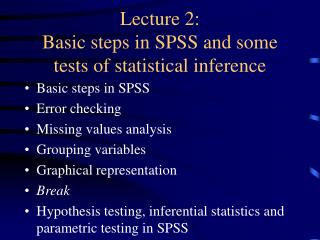DownloadDownload PresentationLecture 2: Basic steps in SPSS and some tests of statistical inference

# Lecture 2: Basic steps in SPSS and some tests of statistical inference

Download Presentation## Lecture 2: Basic steps in SPSS and some tests of statistical inference

- - - - - - - - - - - - - - - - - - - - - - - - - - - E N D - - - - - - - - - - - - - - - - - - - - - - - - - - -
##### Presentation Transcript

1. Lecture 2: Basic steps in SPSS and some tests of statistical inference • Basic steps in SPSS • Error checking • Missing values analysis • Grouping variables • Graphical representation • Break • Hypothesis testing, inferential statistics and parametric testing in SPSS

2. Exercises • Exercise data can be found on the website: www.ex.ac.uk/ebrg

3. Statistical inference: the null and alternative hypotheses (H0,H1) • Statistical inference is concerned with differences between samples / populations • Null hypothesis: “There is no significant difference between A and B” • Alternative hypothesis: “There is a significant difference between A and B”

4. One and Two tailed tests • Very often, we will want to examine the ‘direction’ of a difference • One-tailed test: specifying the direction • Two-tailed test: no direction specified • Alternative hypothesis changes for one-tailed test: • “A is significantly greater or less than B”

5. Determining your data types • Population: all possible cases • Sample: your selection of the population • Tied or ‘paired’ samples: Samples that are linked, perhaps in time (e.g. before/after samples)

6. What type of test is appropriate? • Parametric: Classical tests • Non-parametric: Less powerful tests • Knowing your data

7. Test selection • The choice of statistical test is crucial • Various tests depending on test requirements: Parametric Non-parametric • Test calculation can be undertaken in SPSS

8. Significance testing • How significant is the test result? Does it show a ‘real’ difference, or could it have occurred by chance? • With every test, there will be a probability distribution, which looks like the normal distribution • This distribution specifies the probability of the result (test statistic) occurring by chance or random variation

9. Significance - continued • Probability is measured as 100% = 1.00 • Each test has a critical ‘rejection’ area on its distribution where test statistics must be rejected, as they are too large than to have occurred by chance, according to the number in the sample • Accordingly, all tests have critical values for different levels of significance and differently tailed tests

10. Interpreting significance • To simplify matters, we use significance ‘levels’ to determine if a test is significant • We usually use 0.05 (5%) or you could use 0.01 (1%) • This means that there is a 5% probability that the result occurred by chance, i.e. we can be 95% confident in its importance